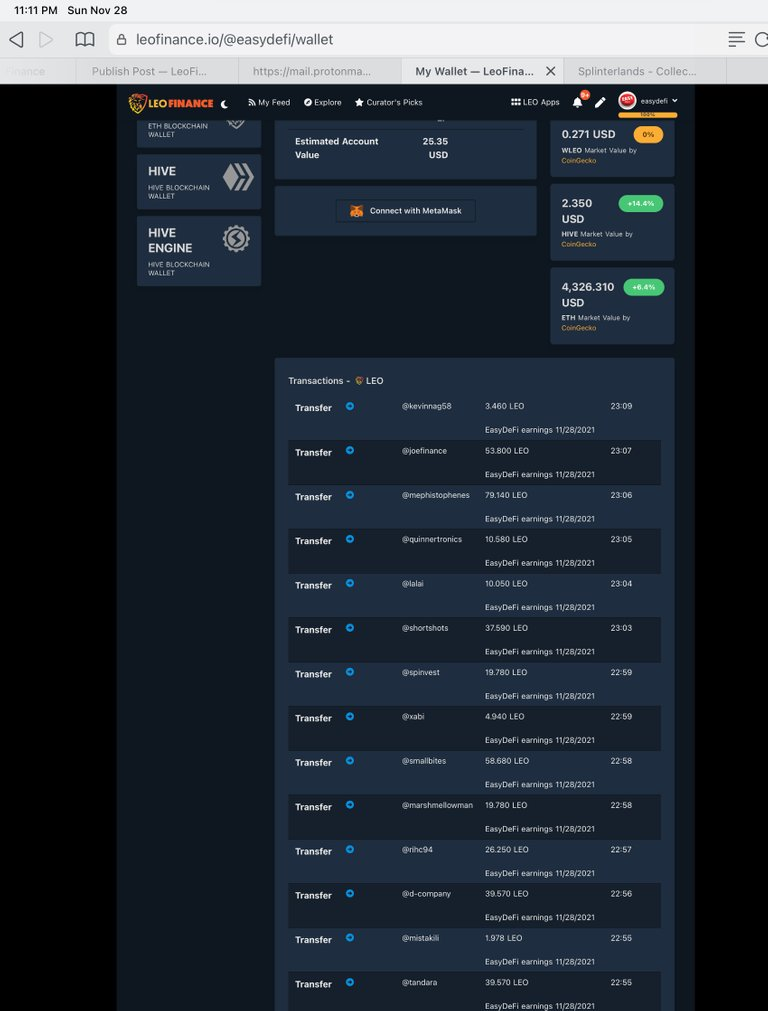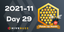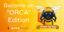# EasyDeFi Investors Report November 28th, 2021(Edited)

# Investors earnings report November 28th, 2021.The pooled Leo is converted to Cub-BUSD, and the earnings for this past week were 205 Cub, which traded for 451 Leo and 45 went to @shortsegments as an Admin Fee. This left 405 BLEO for the stake based investors.

New investments 11/22/21 included today
@kevinnag58 sent 50 Leo
@tandara sent 500 Leo

This weeks Stake based earnings

@joefinance 2720/20,471= 0.133x405=53.8 Leo
@mephistophenes 4000/20,471=0.195x405=79.14 Leo
@quinnertronics 535/20,471=0.0261x405=10.58 Leo
@lalai 508/20,471=0.0248x405=10.05 Leo
@shortshots 1900/20,471=0.0928x405=37.59 Leo
@spinvest 1000/20,471=0.0488=19.78
@xabi 250/20,471=0.01221x405=4.94 Leo
@smallbites 2966/20,471=0.14488x405=58.68 Leo
@marshmellowman 1000/20,471=0.049x405=19.78 Leo
@rihc94 1327/20,471=0.0648x405=26.25 Leo
@d-company 2000/20,471=0.098x405=39.57 Leo
@mistakili 100/20,471=0.00488x405=1.978 Leo
@tandara 2000/20,471=0.098x405=39.57
@kevinnag58 175/20,471=0.008549x405=3.46 Leo

## Estimated Annualized Investment

#### Using ROI=return/investment x 100%

@joefinance 2720 Leo invested, 55 Leo per week times 52 weeks = 2860 Leo earned, or 2860/2720= 1.05 x 100% = 105% one year return!

@mephistophenes 4000 Leo invested, 80 Leo per week earnings x 52 weeks = 4160 Leo, 4160/4000= 1.04 x 100% = 104% return in one year!

@quinnertronics invested 535 Leo, 11 Leo per week earnings x 52 = 572 Leo earned, 572/535= 1.07 x 100= 107% return in one year.
@lalai 508 Leo invested, 10 Leo earned per week, x 52 = 520/508= 1.024x100% = 102.4% return in one year!

@shortshots 1900 Leo invested, 38 Leo per week earned, x 52 weeks= 1976/1900= 1.04x100%= 104% return in one year.

@spinvest 1000 Leo invested, 20 Leo x 52 weeks= 1040 Leo / 1000 Leo = 1.04x100%=104% return in one year.

@xabi 250 Leo invested, 5 Leo per week earned, x 52 weeks= 260 Leo/ 250 Leo= 1.04x100%= 104% return in one year.

@smallbites 2966 Leo invested, 59 Leo per week x 52 = 3068 earned, 3068/2966 invested = 1.034, x 100% = 103.4% annual return.

@marshmellowman 1000 Leo invested, 20 Leo x 52 weeks= 1040 Leo / 1000 Leo = 1.04x100%=104% return in one year.

@rihc94 1327 Leo invested, 26.25 Leo per week earned, x 52 = 1365 Leo earned in one year, 1365/1327- 1.0286x100%= 102.86% return in one year.

@d-company 2000 Leo invested, 40 Leo x 52 weeks= 2080 Leo / 2000 Leo = 1.04x100%=104% return in one year.

@mistakili 100 Leo invested, 2 Leo per week x 52 = 104 Leo earned, 104/100=1.04x 100%=104% return in one year.

@tandara 2000 Leo invested, 40 Leo x 52 weeks= 2080 Leo / 2000 Leo = 1.04x100%=104% return in one year.

@kevinnag58 175 Leo invested, 3.5 Leo per week times 52= 182 Leo / 175 Leo= 1.04x100%=104% return in one year.

Based on earnings over 3 months, I estimate each investor will earn over a 100% ROI for the year, plus an airdrop of Polygon Cub.

A calculation of the estimated airdrop for each investor will be posted later.

#### The Leo went out today, here are the Wallet Transfers.

.@easydefi
.
.
.

EasyDeFi Investors Report November 28th, 2021

Posted Using LeoFinance Beta

0
0
0.000Congratulations @easydefi! You have completed the following achievement on the Hive blockchain and have been rewarded with new badge(s):You distributed more than 50 upvotes.Your next target is to reach 100 upvotes.

If you no longer want to receive notifications, reply to this comment with the word `STOP`Hive Power Up Month - Feedback from Day 29Hive Power Up Day - December 1st 2021 - Become an Orca!